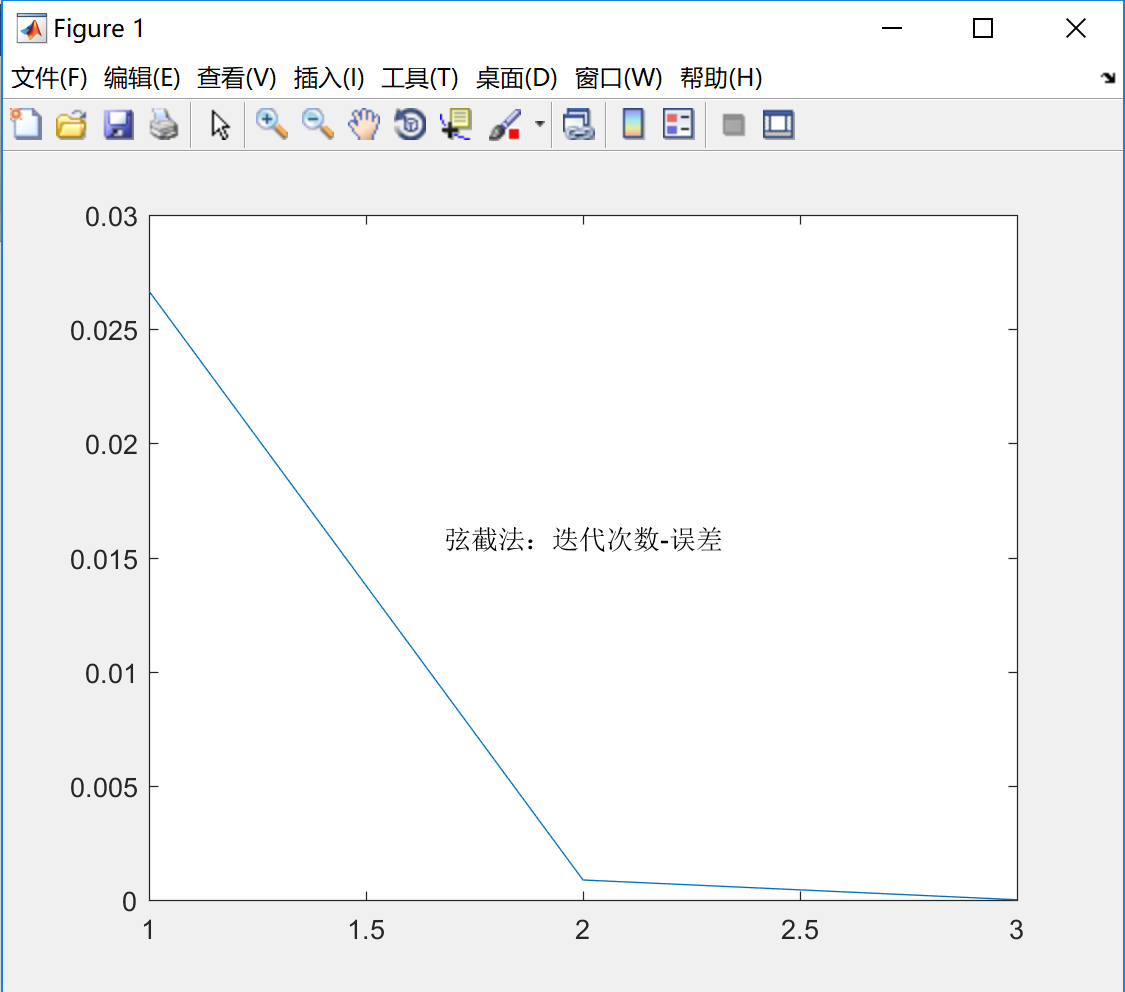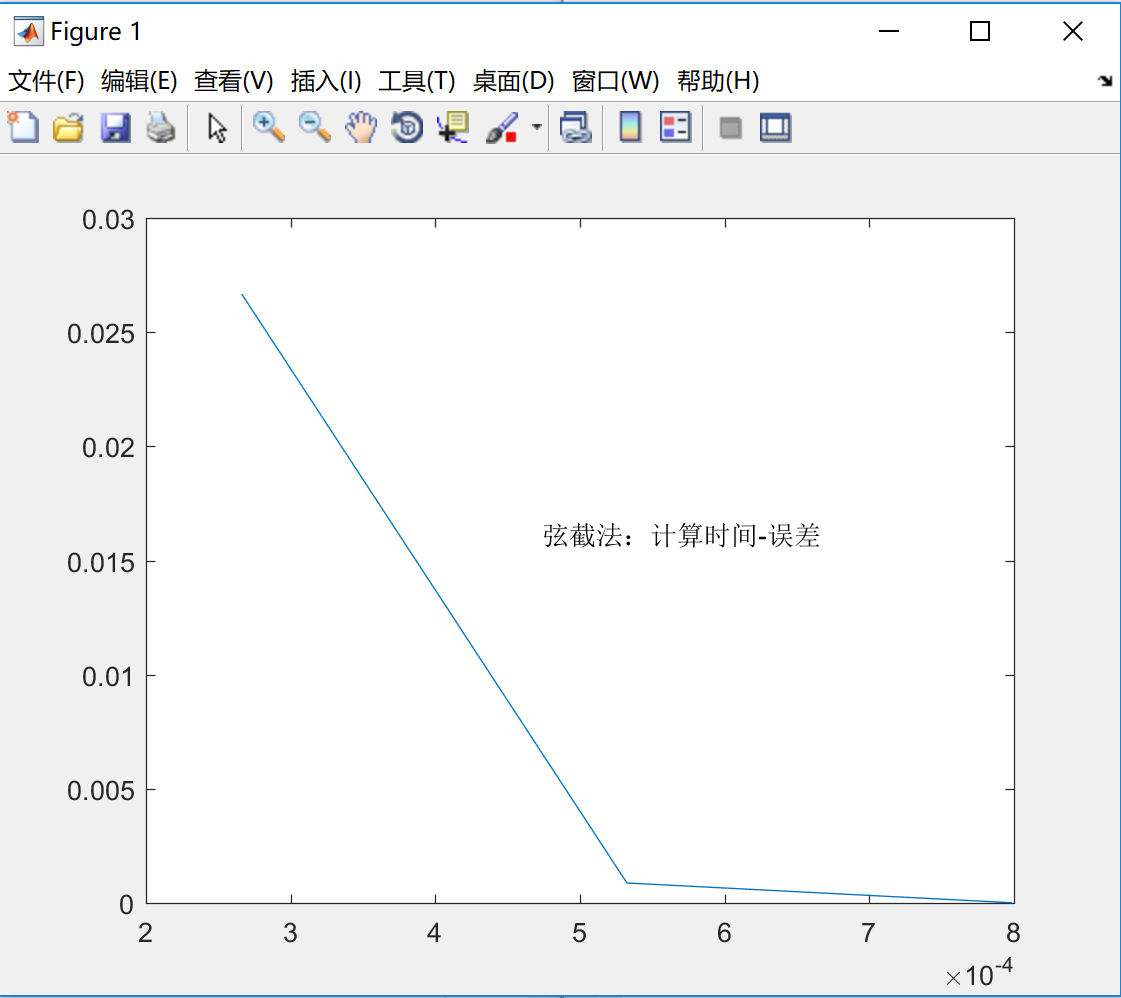# Halo

A magic place for coding

0%

## 介绍

前面提到的简化牛顿法是对牛顿法的一个改进，今天我就来介绍另一个基于牛顿法改进的方法–弦截法。它主要是从插值的角度来对牛顿法进行改进。

## 分析

弦截法也是牛顿法的一个变种，同样也是为了避免计算$f^{‘}(x_k)$。这里采用的方法是使用已求的函数值$f(x_k),f(x_{k-1}),…$来回避导数值$f^{‘}(x_k)$的计算，这种方法是建立在插值原理的基础上的。
设$x_k, x_{k-1}$是$f(x) = 0$的近似根，我们利用$f(x_k),f(x_{k-1})$构造一次插值多项式$p_1(x)$，并用$p_1(x) = 0$的根作为$f(x) = 0$的新的近似根$x_{k+1}$，根据一次插值公式：
$$p_1(x) = f(x_k) + \frac{f(x_k) - f(x_{k-1})}{x_k - x_{k-1}}(x - x_k)，$$

$$x_{k+1} = x_k - \frac{f(x_k)}{f(x_k) - f(x_{k-1})}(x_k - x_{k-1})，$$

弦截法的几何意义是，使用曲线$y = f(x)$上的两点$x_k, x_{k-1}$的弦线与$x$轴的交点作为作为$x^*$的近似解。弦截法与牛顿法都是线性化方法，但是两者有很大不同。牛顿法在计算$x_{k+1}$的时候只用到了上一步的结果$x_k$，而弦截法在求$x_{k+1}$时要用到前两部的计算结果$x_k$，$x_{k-1}$，因此在使用弦截法的时候要首先给出两个值$x_0$，$x_1$。

Matlab代码：

## 小结

对上述实现的代码进行简单的数值实验，结果如下：弦截法在计算下一步的值的时候需要用到前两步的结果，其收敛速度虽然没有达到牛顿法的平方收敛，但是也是相当快的。
关于弦截法的分析与实现就到这里了，谢谢！

#### 参考资料：

1.数值分析（第5版） 李庆扬，王能超，易大义

Welcome to my other publishing channels